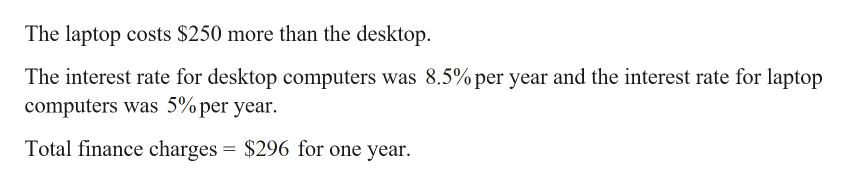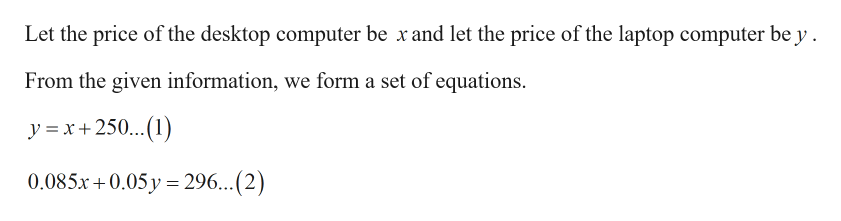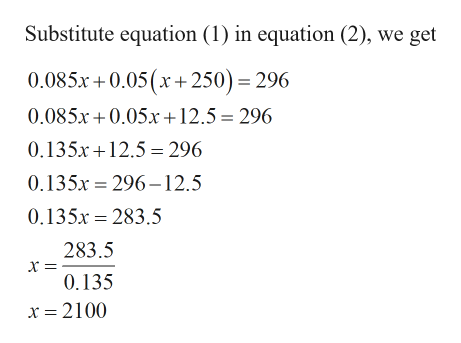# Trey bought a desktop computer and a laptop computer. Before finance charges, the laptop cost \$250 more than the desktop. He paid for the computers using two different financing plans. For the desktop the interest rate was 8.5% per year, and for the laptop it was 5% per year. The total finance charges for one year were \$296. How much did each computer cost before finance charges?

Question
27 views

Trey bought a desktop computer and a laptop computer. Before finance charges, the laptop cost \$250 more than the desktop. He paid for the computers using two different financing plans. For the desktop the interest rate was 8.5% per year, and for the laptop it was 5% per year. The total finance charges for one year were \$296. How much did each computer cost before finance charges?

check_circle

Step 1

Given:help_outlineImage TranscriptioncloseThe laptop costs \$250 more than the desktop. The interest rate for desktop computers was 8.5% per year and the interest rate for laptop computers was 5% per year. Total finance charges = \$296 for one year. fullscreen
Step 2

Calculation:help_outlineImage TranscriptioncloseLet the price of the desktop computer be x and let the price of the laptop computer be y . From the given information, we form a set of equations. y = x +250...(1) 0.085x + 0.05 y = 296...(2) fullscreen
Step 3help_outlineImage TranscriptioncloseSubstitute equation (1) in equation (2), we get 0.085x +0.05(x +250) = 296 0.085x + 0.05xr+12.5 = 296 0.135x+12.5 = 296 0.135x = 296-12.5 0.135x = 283.5 283.5 0.135 x = 2100 fullscreen

### Want to see the full answer?

See Solution

#### Want to see this answer and more?

Solutions are written by subject experts who are available 24/7. Questions are typically answered within 1 hour.*

See Solution
*Response times may vary by subject and question.
Tagged in

### Other